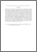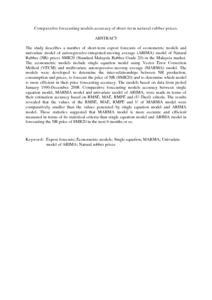# Comparative forecasting models accuracy of short-term natural rubber prices.

## Citation

Aye, Aye Khin and Mohamed, ZainalAbidin and Shamsudin, Mad Nasir (2011) Comparative forecasting models accuracy of short-term natural rubber prices. Trends in Agricultural Economics , 4 (1). pp. 1-17. ISSN 1994-7933; ESSN:2077-2246

## Abstract

The study describes a number of short-term expost forecasts of econometric models and univariate model of autoregressive-integrated-moving average (ARIMA) model of Natural Rubber (NR) prices SMR20 (Standard Malaysia Rubber Grade 20) in the Malaysia market. The econometric models include single equation model using Vector Error Correction Method (VECM) and multivariate autoregressive-moving average (MARMA) model. The models were developed to determine the inter-relationships between NR production, consumption and prices, to forecast the price of NR (SMR20) and to determine which model is more efficient in their price forecasting accuracy. The models based on data from period January 1990-December 2008. Comparative forecasting models accuracy between single equation model, MARMA model and univariate model of ARIMA, were made in terms of their estimation accuracy based on RMSE, MAE, RMPE and (U-Theil) criteria. The results revealed that the values of the RMSE, MAE, RMPE and U of MARMA model were comparatively smaller than the values generated by single equation model and ARIMA model. These statistics suggested that MARMA model is more accurate and efficient measured in terms of its statistical criteria than single equation model and ARIMA model in forecasting the NR price of SMR20 in the next 6 months or so.Preview
PDF (Abstract)
Comparative forecasting models accuracy of short.pdfView Item